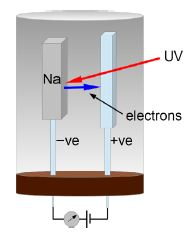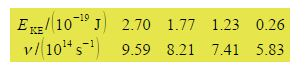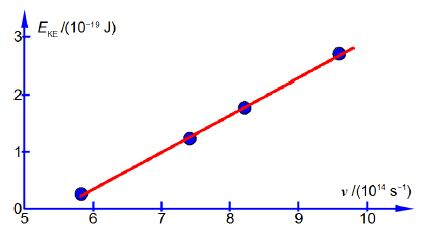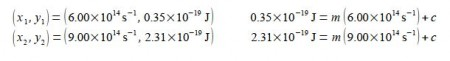Chemistry

# Explain Photoelectric Experimental Results

Light of various selected frequencies v was directed at a sodium metal target inside a vacuum system. The kinetic energy of the ejected electrons EKE was measured by the voltage required to stop them, this stopping voltage being then converted to units of joules. Below Figure is a schematic drawing of the experiment, it does not show the grid electrode to which the stopping voltage is applied for reasons of clarity.There is a minimum energy of the UV photon which must be used for a given metal for it to lose an electron, this minimum energy is called the “work function” of the metal ϕ (upper case phi) the work function is the solid state equivalent to the ionization energy of a gas phase atom. The UV photon is annihilated when it is absorbed and its energy by is used to overcome the work function and eject the electron with any excess energy from the original photon appearing as the ejected electron’s kinetic energy EKE

hv = ϕ + EKE

A student took these experimental measurements of the photoelectric effect for sodium.Firstly, manually plot the graph of EKE against the v (this is good practice for exams!). Secondly, does the data form a good straight line plot, i.e. is the energy of the ejected electrons proportional to the frequency of the light hitting the sodium metal target? Thirdly, determine the values of the gradient of the line, Planck’s constant h. Fourthly, determine the work function ϕ from the x-axis intercept (not the y-axis intercept).There is a linear dependence of the kinetic energy of the ejected electron with the frequency of the light. There is a threshold frequency for the photoelectric effect. To find the equation of the line y = mx + c by hand, we need to pick two points on the line and use their (x, y) coordinates. Do not use the data points themselves else you are wasting your time drawing a graph! The two points of the line that I measured from the full sized manually drawn graph lead to the two simultaneous equations below.Subtracting the two simultaneous equations gives

1.96 x 10-19 J = m (3 x 1014 s-1);    m = 6.53 x 10-34 J s

The student’s value for Planck’s constant from the gradient is: h = 6.53 x 10-34 J s

The accepted value for Planck’s constant is h = 6.626x 10-34 J to four significant figures. Adding the two simultaneous equations and using the student’s value for m the student found the intercept

2.66 x 10-19 J = (6.53 x 10-34 J s)(15.00 x 1014 s-1) +2c ;      c = —3.57 x 10-19 J

The student’s equation of the straight line is

EKE = (6.53 x 10-34 J s) v — (3.57 x 10-19 J)

The work function is the minimum photon energy required for electron emission, ϕ = hv0

ϕ = 6.53 x 10-34 J s) (5.47 x 1014 s-1) = 3.57 x 10-19 J

The accepted value for the work function of sodium metal is ϕ = 338 x 10-19 J, so the student’s work function is inaccurate by about 6% and needs some improvement to either the apparatus or method.